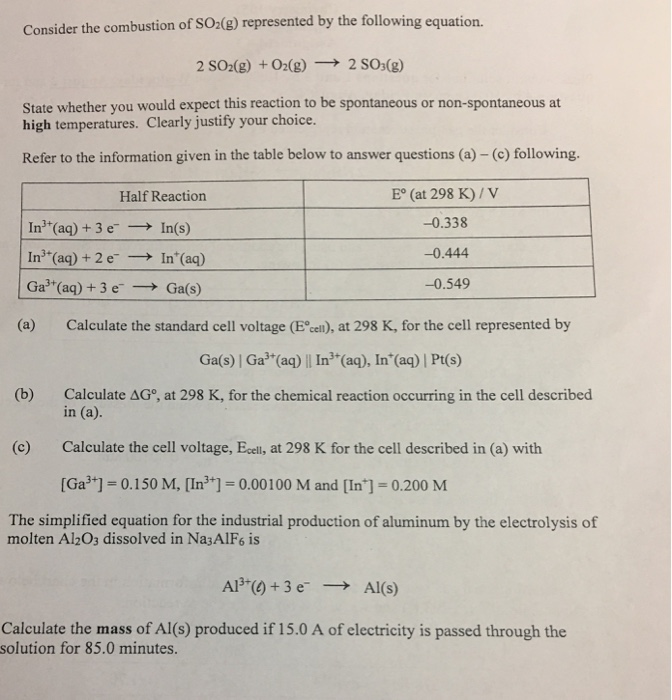# Question & Answer: Consider the combustion of SO_2(g) represented by the following equation. 2SO_2(g) +…..Consider the combustion of SO_2(g) represented by the following equation. 2SO_2(g) + O_2(g) rightarrow 2 SO_3(g) State whether you would expect this reaction to be spontaneous or non-spontaneous at high temperatures. Clearly justify your choice. Refer to the information given in the table below to answer questions (a) – (c) following. Calculate the standard cell voltage (E degree _cell), at 298 K, for the cell represented by Ga(s) | Ga^3+ (aq) || In^3+ (aq), In^+ (aq) | Pt(s) Calculate Delta G degree, at 298 K, for the chemical reaction occurring in the cell described in (a). Calculate the cell voltage, E_cell, at 298 K for the cell described in (a) with [Ga^3+] = 0.150 M, [In^3+] = 0.00100 M and [In^+] = 0.200 M The simplified equation for the industrial production of aluminum by the electrolysis of molten Al_2O_3 dissolved in Na_3AlF_6 is Al^3+(l) + 3e^- rightarrow Al(s) Calculate the mass of Al(s) produced if 15.0 A of electricity is passed through the solution for 85.0 minutes.

2SO2(g) + O2(g) —-> 2so3(g)

DH0rxn = 2*-395.7-(2*-296.81+1*0) = -197.78 kj

Don't use plagiarized sources. Get Your Custom Essay on
Question & Answer: Consider the combustion of SO_2(g) represented by the following equation. 2SO_2(g) +…..
GET AN ESSAY WRITTEN FOR YOU FROM AS LOW AS \$13/PAGE

DS0rxn = 2*256.7-(2*248.1+1*205) = -187.8 j/k

DG0 = dh0-Tds0

= -197.78-(273.15*-187.8)

= 51.1 kj

as DG0 = +ve , it is nonspontaneous at standrad temperature and higher also.

a) E0cell = E0cathode – E0anode

= -0.444 -(-0.549)

= 0.105 v

b) 2Ga(s) + 3In^3+(aq) —-> 3In^+(aq) + 2Ga^3+(aq)

DG0 = – nFE0cell

= -6*96500*0.105

= -60795 joule

c) Ecell = E0cell – 0.0591/nlog[Ga^3+]^2[In^+]^3/[In^3+]^3

= 0.105 – (0.0591/6)log(0.15^2*0.2^3/0.001^3)

= 0.0532 V